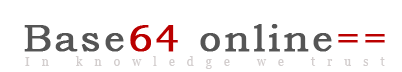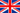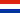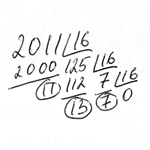Base64 decode | Decimal to binary | Hexadecimal converter | Hash generator | Colored QR Code
Cisco password cracker | Base64 to image | MAC address database| Online Device Explorer
Decode gzinflate base64 | IP Address | TCP-UDP Port check | Cisco docs

# Binary, octal, decimal, hexadecimal converter.

Online tool to convert decimal to hexadecimal, binary and octal representation.

Decimal (Base 10)

Binary (Base 2)

Octal (Base 8)

## Manually converting decimal to hex.

To convert decimal to hexadecimal manually you must follow the next steps. The table below represents hexadecimal equivalent of the decimal characters.

 Decimal 0 1 2 3 4 5 6 7 8 9 10 11 12 13 14 15 Hexadecimal 0 1 2 3 4 5 6 7 8 9 A B C D E F
 For example we will convert decimal 2011 into hexadecimal. We must divide 2011 by 16. The result will be 125 and remainder 11. Next, we must divide 125 by 16. The result is 7 and the remainder is 13. Next, divide 7 by 16. The result is zero and the remainder is 7. Now write down the remainders beginning with next one. We got 7, 13 and 11. From the table above we know that 7 is equal to hexadecimal 7, 13 is equal to hexadecimal D and 11 is equal to hexadecimal B. So, 2011 converted into hexadecimal will be 7DB, 2012 in hexadecimal will be 7DC and 2042 will be 7FA. 3,221,225,705 will be 0xC00000E9 or just C00000E9.Online decode encode tools:

Base64 decode | Decimal to binary | Hexadecimal converter | Hash generator | QR Code
Cisco password cracker | Base64 to image | MAC address database | Online Device Explorer
Decode gzinflate base64 | IP Address | TCP-UDP Port check | Books | Hashes